• 问答
• 技术
• 实践
• 资源

本文目录

40 EDT：用于底层视觉的高效图像处理 Transformer
(来自 港中文，思谋科技)
40.1 EDT 原理分析
40.2 EDT 代码解读

Transformer 是 Google 的团队在 2017 年提出的一种 NLP 经典模型，现在比较火热的 Bert 也是基于 Transformer。Transformer 模型使用了 Self-Attention 机制，不采用 RNN 的顺序结构，使得模型可以并行化训练，而且能够拥有全局信息。

40 EDT：用于底层视觉的高效图像处理 Transformer

https://arxiv.org/abs/2112.10175

EDT 模型架构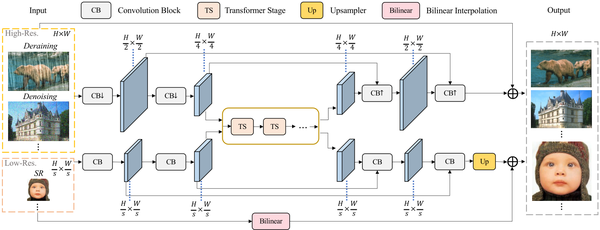Shifted Crossed Local Attention

Shifted Crossed Local Attention 的过程如下图2所示，给定输入特征 $\mathbf{X} \in {\mathbb R}^{(H \times W) \times C}$ ，把它按照 channel 维度分成均等的2份，分别执行水平和垂直的 Window-based Attention，Window size 分别是 $(h, w)$ 和 $(w, h)$ 。最后把这两部分的结果 concat 在一起。写成公式就是：

\begin{aligned} &\mathbf{X}=\left[\mathbf{X}{1}, \mathbf{X}{2}\right], \text { where } \mathbf{X}{1}, \mathbf{X}{2} \in \mathbb{R}^{(H \times W) \times C / 2}, \ &\mathbf{X}{1}^{\prime}=\mathrm{H}-\mathrm{MSA}\left(\mathbf{X}{1}\right), \ &\mathbf{X}{2}^{\prime}=\operatorname{V-MSA}\left(\mathbf{X}{2}\right), \ &\text { (S)CL-MSA }(\mathbf{X})=\operatorname{Proj}\left(\left[\mathbf{X}{1}^{\prime}, \mathbf{X}{2}^{\prime}\right]\right), \end{aligned} \tag{1}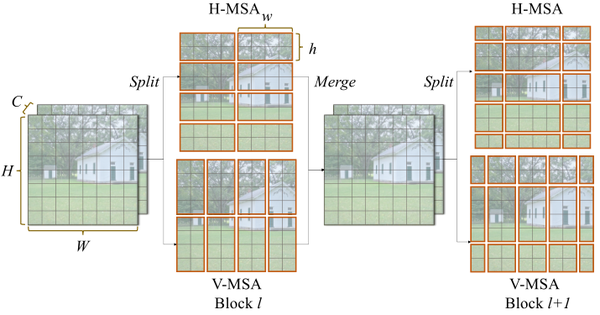Shifted Crossed Local Attention 的计算复杂度为：

$$\Omega((\mathrm{S}) \mathrm{CW}-\mathrm{MSA})=4 H W C^{2}+2 h w H W C \tag{2}$$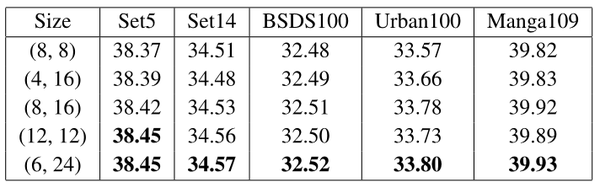Anti-Blocking FFN

\begin{aligned} &\mathbf{X}^{\prime}=\operatorname{Act}(\operatorname{Linear}(\mathbf{X})) \ &\mathbf{X}=\operatorname{Linear}\left(\operatorname{Act}\left(\operatorname{Anti-Block}\left(\mathbf{X}^{\prime}\right)\right)\right) \end{aligned} \tag{3}

Anti-blocking 操作是一个 5×5 的 Depthwise Convolution。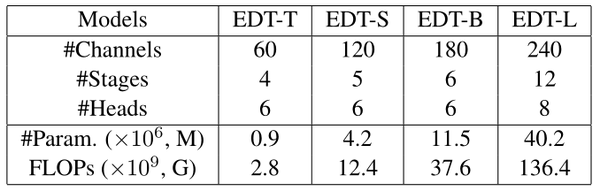Centered Kernel Alignment 研究隐藏层的表示相似性

• 基于点积的相似性度量 (Dot Product-Based Similarity)：

$$\left\langle\operatorname{vec}\left(X X^{\mathrm{T}}\right), \operatorname{vec}\left(Y Y^{\mathrm{T}}\right)\right\rangle=\operatorname{tr}\left(X X^{\mathrm{T}} Y Y^{\mathrm{T}}\right)=\left|Y^{\mathrm{T}} X\right|_{\mathrm{F}}^{2} \tag{S.1}$$

• 基于 Hilbert-Schmidt 独立性的度量 (Hilbert-Schmidt Independence Criterion)：

$$\frac{1}{(m-1)^{2}} \operatorname{tr}\left(X X^{\mathrm{T}} Y Y^{\mathrm{T}}\right)=\left|\operatorname{cov}\left(X^{\mathrm{T}}, Y^{\mathrm{T}}\right)\right|_{\mathrm{F}}^{2} \tag{S.2}$$

\begin{aligned} \frac{1}{(m-1)^{2}} \operatorname{tr}\left(X X^{\mathrm{T}} Y Y^{\mathrm{T}}\right) &=\frac{1}{(m-1)^{2}} \operatorname{tr}\left(\left(Y^{\mathrm{T}} X\right)^{T} Y^{\mathrm{T}} X\right) \ &=\frac{1}{(m-1)^{2}} \sum{i=1}^{p} \sum{j=1}^{p}\left(Y^{\mathrm{T}} X{i, j}\right)\left(Y^{\mathrm{T}} X{i, j}\right) \ &=\frac{1}{(m-1)^{2}} \sum{i=1}^{p} \sum{j=1}^{p}\left(Y^{\mathrm{T}} X{i, j}\right)^{2} \ &=\frac{1}{(m-1)^{2}} \operatorname{cov}\left(X^{\mathrm{T}}, Y^{\mathrm{T}}\right) {i, j}^{2} \ &=\left|\operatorname{cov}\left(X^{\mathrm{T}}, Y^{\mathrm{T}}\right)\right|_{\mathrm{F}}^{2} \end{aligned} \tag{S.3}

$$\operatorname{HSIC}(K, L)=\frac{1}{(m-1)^{2}} \operatorname{tr}(K H L H) \tag{S.4}$$

• CKA 度量 (Centered Kernel Alignment)：

$$\operatorname{CKA}(K, L)=\frac{\operatorname{HSIC}(K, L)}{\sqrt{\operatorname{HSIC}(K, K) \operatorname{HSIC}(L, L)}} . \tag{5}$$

HSIC 对各向同性标度是变化的，但可以通过归一化使其不变。给定 $m$ 个数据点，得到两个层的输出 $X \in \mathbb{R}^{m \times p{1}}$ 和 $Y\in \mathbb{R}^{m \times p{2}}$ ，其中 $p_1$ 和 $p_2$ 分别代表了输出特征的神经元数量。

EDT 通过格莱姆矩阵 $K=XX^\top$ 和 $L=YY^\top$ 来计算 CKA。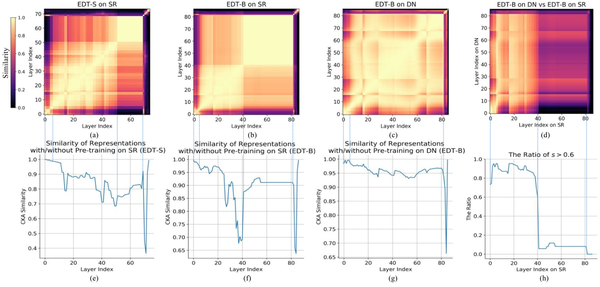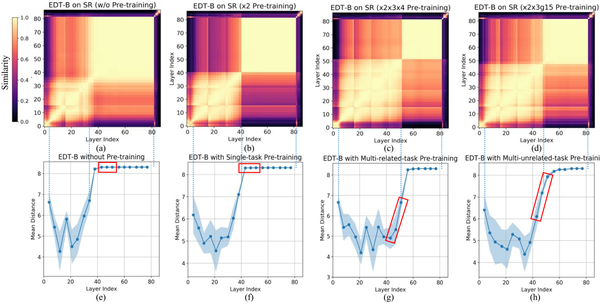• 超分模型表现出明显的阶段性特征，各阶段的比例随模型尺寸的变化而变化，去噪模型呈现相对均匀的结构。
• 去噪模型层与超分模型的低层更加相似，包含更多的局部信息。
• 单任务预训练主要影响超分模型的高层，但对去噪模型影响有限。
• SR 模型表示在低层中包含更多的局部信息，与之相对应的 Transformer 块的 attention head 的平均距离较小；在高层中包含更多的全局信息，与之相对应的 Transformer 块的 attention head 的平均距离较大。
• 与单任务预训练相比，相关多任务预训练 (图(g)) 将更多的第3个块的全局表征转化成了局部表征，增加了第2块的范围。不相关多任务预训练 (图(h)) 的这种现象的表现偏弱。
• 3种预训练方法都可以通过将不同程度的局部信息作为一种归纳偏差引入模型的中间层，从而大大提高模型的性能，其中相关多任务的预训练效果最好。

• single task：指针对某一特定任务 (如×2 SR) 进行单一模型的训练。
• related tasks：对高度相关的任务 (如×2，×3，×4 SR) 训练单一模型。
• unrelated tasks：对不相关的任务(如×2，×3SR, level-15 去噪) 训练单一模型。

Classical SR 实验结果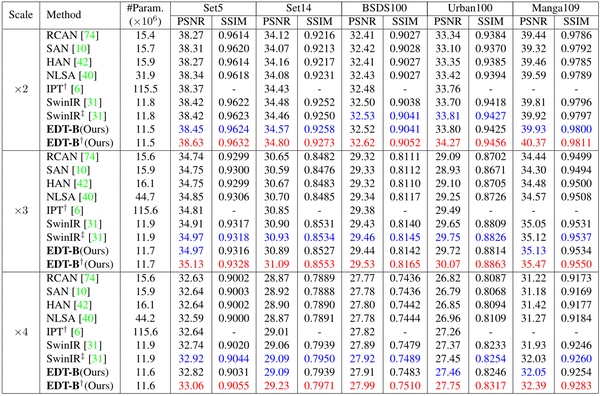Lightweight SR 实验结果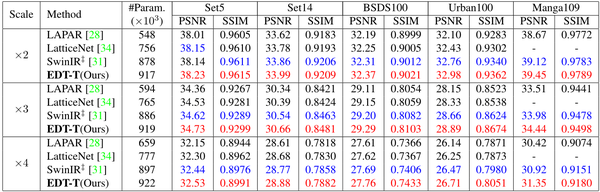Denoising 实验结果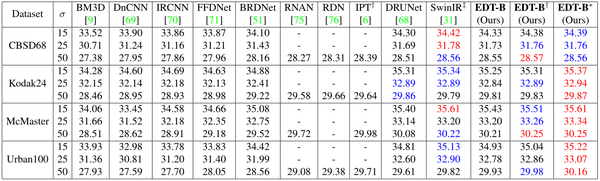Deraining 实验结果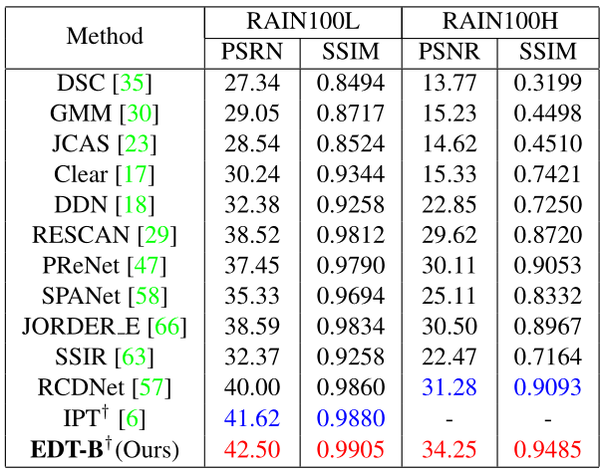总结

•0
•0
•235收藏

• 45

关注
• 138

获赞
• 9

精选文章

• 人脸识别方向

• 人脸识别

• 684
• 104
• 352
• 160
• 182